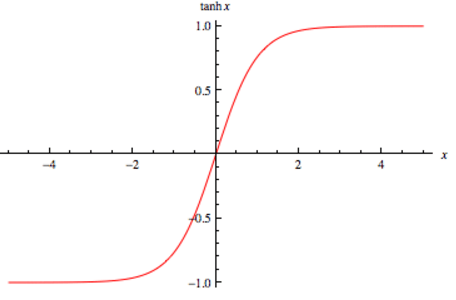/  Deep Learning Interview questions and answers   /  Explain Hyperbolic Tangent or Tanh, ReLU (Rectified Linear Unit)## Explain Hyperbolic Tangent or Tanh, ReLU (Rectified Linear Unit)

### (a) Hyperbolic Tangent or Tanh

Ans: The Activation Function which is better than Sigmoid Function is Tanh function which is also called as Hyperbolic Tangent Activation Function. Similar to Sigmoid Function it is also have simple Derivative function. Range of values of Tanh function is from -1 to +1. It is of S shape with Zero centered curve.

Due to this, Negative inputs will be mapped to Negative, zero inputs will be mapped near Zero. Tanh function is monotonic that is it neither increases nor decreases while its derivative is not monotonic.Hyperbolic Tangent Function: tanh(x) = (ex – e-x) / (ex + e-x)

Tanh Function can also be derived from Sigmoid Function

```f(x) = tanh(x) = 2/(1 + e-2x) - 1

OR

tanh(x) = 2 * sigmoid(2x) - 1```

### (b) ReLU (Rectified Linear Unit):

ReLU is the short form of Rectified Linear Activation Unit Function which is most widely used in Neural Network. It is mainly implemented in Hidden layers of Neural Network. For Zero inputs and Negative inputs it will give output as Zero where as for positive input it will give the same positive output. Range of ReLU Function is from 0 to infinity. The Function and its derivative both are monotonic in nature. It is not Zero centered Activation Function as that of Tanh Function.